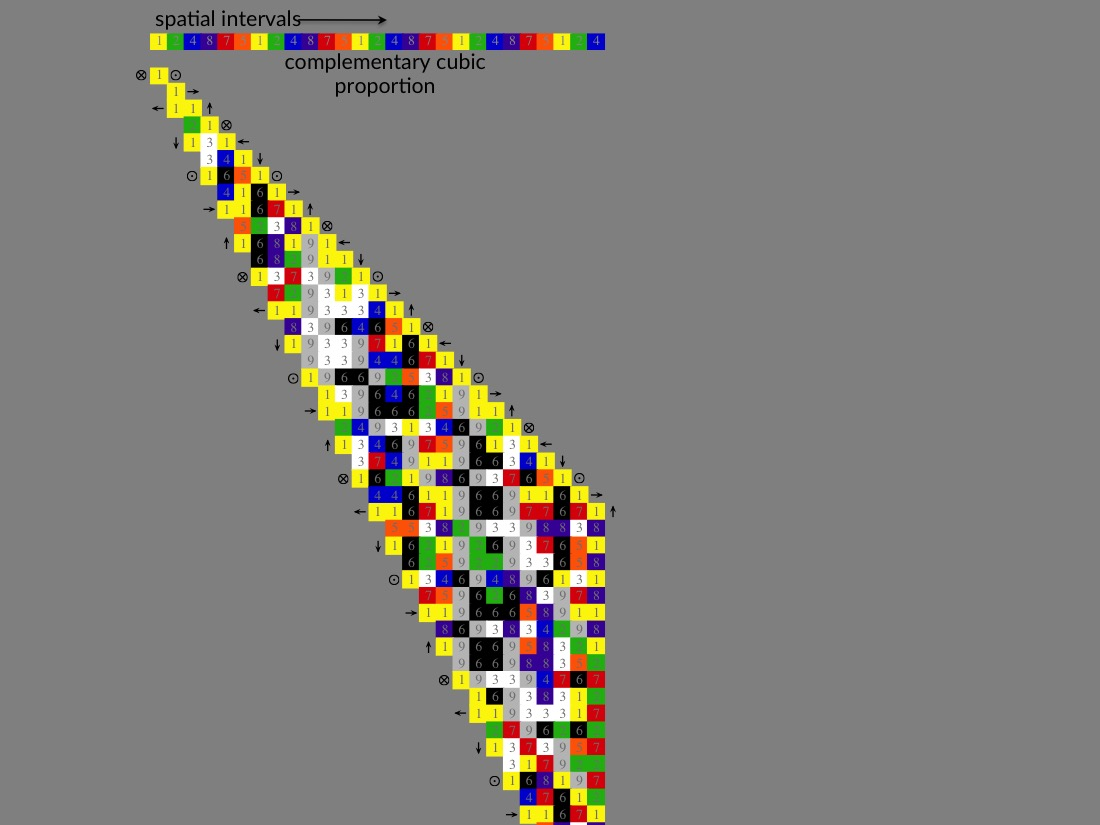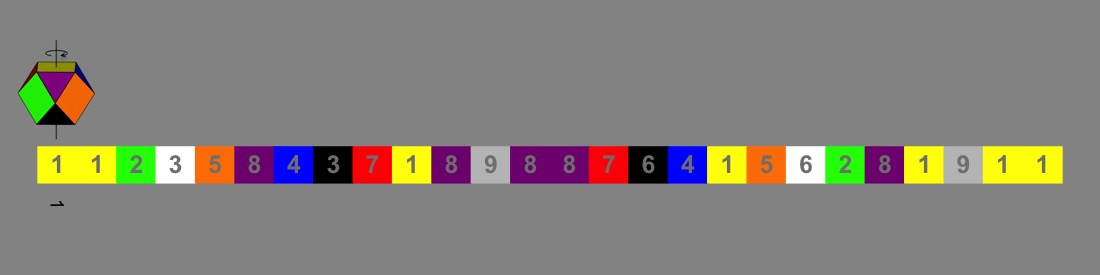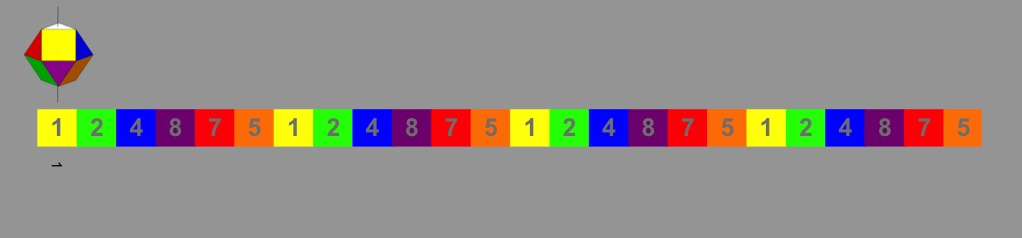And the Pythagoreans say that there is void, ... and this void defines the nature of things, inasmuch as it is a certain separation and definition of things that lie together; and this is true first in the case of numbers, for the void defines the nature of these.
Aristotle
Welcome to the Natural Number interactive page. You’ll find many of the same figures as the animation page, but here you can study them at you pace by manipulating them with your mouse. These visualization aids underscore essential elements of dimensional number theory. Explore the unified spatial/temporal dimensional geometry of Pascal’s triangle as it evolves through the rotational symmetry transformations of its continuous golden proportion (the Fibonacci series) — transformations revealed through analysis of the underlying complementary modulus 9 number symmetry. For more complete treatment of these fundamental natural numeric phenomena explore chapters 3 thru 9 in conjunction with this page.
Spherical symmetry and the Complementary Cuboctahedron…
Use the tabs to toggle between spherical and complementary cuboctahedral geometries in adjacent 3D model overlay. This model is introduced in Chapter 5 with Figure 11.
This natural complementary numeric dimensional symmetry is coordinated about the central sphere or monad at the origin of Pascal’s triangle. It governs the evolution of the continuous golden proportion of 2:1 diagonals (Fibonacci series: 0, 1, 1, 2, 3, 5, 8, …) through rotational symmetry transformations about the 1-8 cubic dipole axis — see Golden Proportion.
Orient the model to the complementary 1-8 cubic dipole axis (opposing square yellow-1 and violet-8) and look for the 4-fold octahedral transformation segments shown in Figure 27 (Chapter 8). Start with the 2-3-5-8 segment by finding the triangular 2-face then rotate and switch hemisphere to the 3-face then rotate and switch hemisphere to the 5-face and again to the 8-face. Rotation through the full series requires two complementary cuboctahedrons: the adjacent spatially polarized one and a temporally polarized one — see Golden Proportion below.

### Complementary

This same symmetry also mediates the evolution of the Lucas continuous golden proportion (2, 1, 3, 4, 7, 11, …) through rotation about the 7-2 dipole axis. Orient the adjacent model to the complementary 7-2 cubic dipole axis and look for the 4-fold octahedral transformation segments shown in Figure 29 (Chapter 8).
The model also supports the subordinate complementary cubic rotational symmetry that mediates transformations of the continuous geometrical proportion (corresponding to succeeding 1:1 diagonals). Explore this with the adjacent model through 6-fold rotation about the 3-6 dipole axis and Figure 24 (Chapter 8) as well as by having across the interactive graphic in the page header.
Triangular Number, mod 9, and the close packing of concentric spheres…
Hover from left to right over the adjacent graphic. Explore the geometric relationship between the triangular number series and modulus 9 number symmetry:
The number 1 and every third triangular number thereafter form the 1+9+18+27+...(9n) additive series (1, 10, 28, 55, …). Each number in this additive series, aside from the first, is a multiple of 9. A concentric triangular arrangement of first 9, then 18, then 27 spheres (9n) about the first central sphere (first 1). This is why in modulus 9 all the numbers in this subset of ‘’1-like’’ triangles reduce to 1 (i.e. 1, 1, 1, 1, … mod 9).
The number 3 and every third triangular number thereafter form the 3+12+21+30+...(3+9n) additive series (6, 21, 45, 78, …). A concentric triangular arrangement of first 12, then 21, then 30 spheres (3+9n) about the first 3 central spheres. In modulus 9 the subset of these ‘’3-like” triangles alternate 3-6-9 (i.e. 3, 6, 9, 3, 6, 9, …) because each number in the additive series reduces to 3.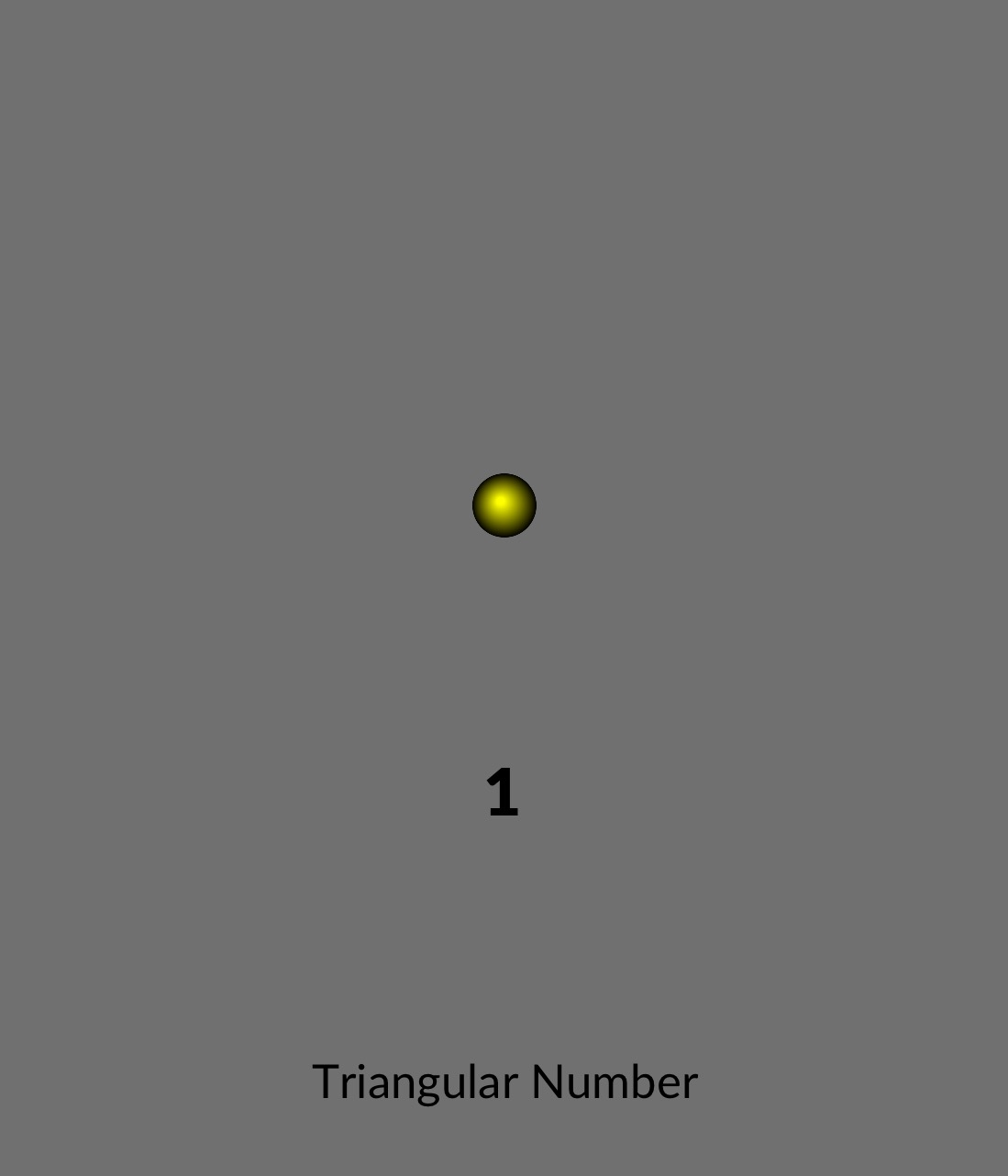The number 6 and every third triangular number thereafter form the 6+15+24+33+...(6+9n) additive series (3, 15, 36, 66, …), which corresponds to the concentric triangular arrangement of first 15, then 24, then 33 spheres (6+9n) about the first 6 central spheres. In modulus 9 the subset of these ‘’6-like’’ triangles alternate 6-3-9 (i.e. 6, 3, 9, 6, 3, 9, …) because each number in the additive series reduces to 6.
Interleaved, these three triangular spherically close-packed planes form the continuous tetrahedral proportion, where the central sphere of 1-like triangles is cuboctahedrally coordinated by 12 surrounding spheres — hinting at its underlying complementary rotational symmetry.
That number admits nothing beyond the ennead (nine), but rather everything circles around within it, is clear from the so-called recurrences: there is natural progression up to it, but after it there is repetition. …so that it is by no means possible for there to subsist any number beyond nine elementary numbers.
Iamblichus
Pascal’s triangle, dimensional invariance, mod 9 and translational proportion…
Hover from left to right across the graphic below to observe the underlying dimensional symmetry of Pascal’s triangle evolve. This figure is meant to accompany the content in Chapters 3 thru 6 of Natural Number.
The geometric constructs in the graphic demonstrate how succeeding columns and rows (translational proportion) are formed by summing their respective predecessor column or row at right angle. The figure also demonstrates the inverse (spatial vs. temporal) relationship between columns and rows.
Chapter 3 of Natural Number provides a geometric explanation for these relationships tied to the interplay of the germinal symmetry elements of point (1) and void (0) that sum as orthogonal vectors to perpetuate the continuous equal proportion of the singular dimensions of space and time.
Chapter 5 ties these succeeding sums of point and void to the incremental incorporation of modulus 9 numeric invariance within succeeding columns and rows, as evidenced by the incremental incorporation neutral 9s within each successor.
Taken together with the relative orientations of linear, triangular, and tetrahedral translational proportion, and the underlying geometric incorporation of nines, all observations are mutually consistent. This points to a repeating pattern of rotation between dimensional orientations, and a continued proportion may be characterized by a minimum of three terms: past, present, and future — thus establishing the dimensional continuum.…there are some who say that there is a right and left of the heavens, as, for instance, those that are called Pythagoreans (for such is their doctrine).
Aristotle
Super-symmetric rotational proportion…
Hover from left to right across the graphic below to observe the complementary rotational symmetry transformations that accompany the continuous proportion of the diagonal intervals of Pascal’s triangle (Note: mirror temporal and temporal/spatial orientations not shown). These graphics are meant to accompany the content in Chapter 8 of Natural Number.
First tab: The continuous Geometrical Proportion of 1:1 diagonal sums (1, 2, 4, 8, 16, 32, …) is shown conforming to complementary cubic symmetry transformations — Transformations between complementary cube faces through rotation about the 3-6 cube-diagonal dipole axis of the complementary color cube. A super-symmetry coordinated about the central spherical point or monad — the singular dimension. Note that this continuous cubic geometry is consistent with the relative orientation of dimensional constraints shown in the preceding example.
Second tab: The continuous Golden Proportion of 2:1 diagonal sums (1, 1, 2, 3, 5, 8, …) is shown conforming to complementary cuboctahedral symmetry transformations. Transformations between complementary octahedral faces through rotation about the octahedral-diagonal dipole axis corresponding to 1-8 cube faces of the complementary cuboctahedron. The continuous golden proportion can be considered the governing proportion of the field due to a symmetric hierarchy and sequential ordering of dimensional invariance that by nature requires only the symmetric intent of point (1) and void (0) to establish the continuum — the minimum number terms required to establish the continued proportion of the unified field. This governing spatial/temporal rotational super symmetry is coordinated about the central singular dimension and is the origin of the natural numeric order of the universe — the virtual dimensional symmetry of energy.

### Geometrical Proportion (complementary cubic)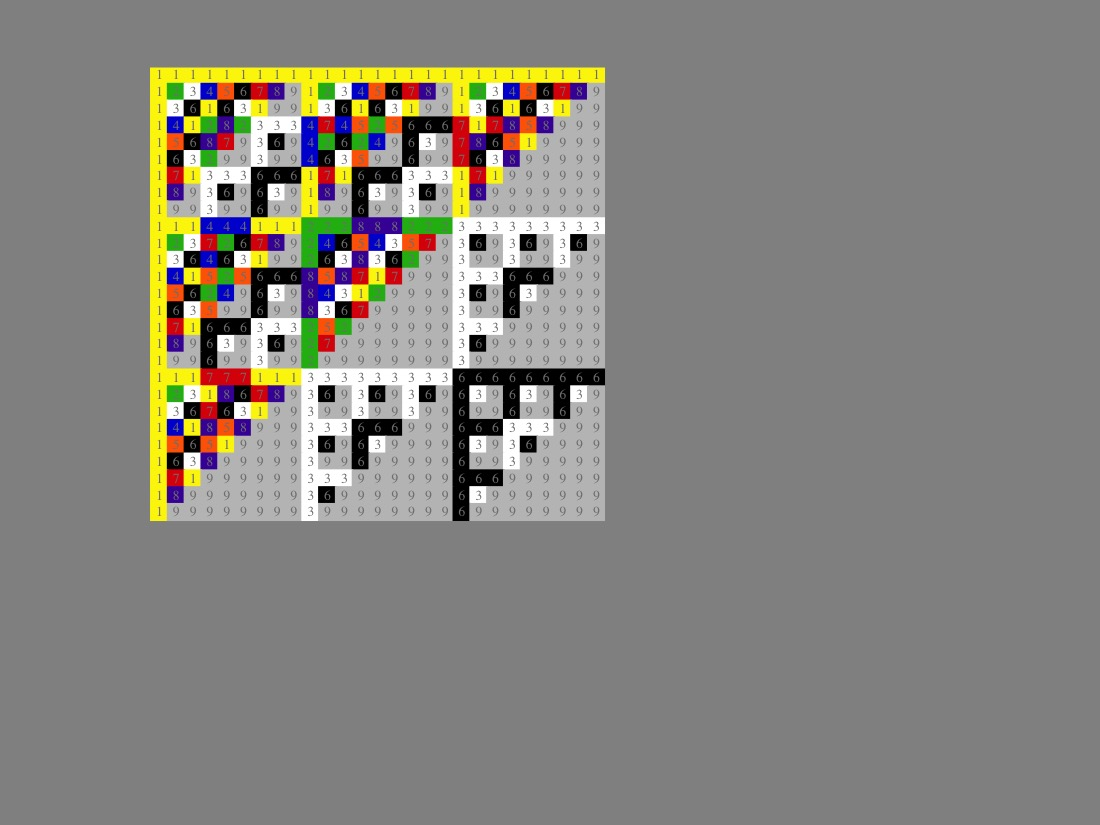### Golden Proportion (complementary cuboctahedral)